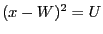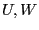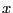Next: Box reduction Up: Filtering Previous: Filtering   Contents

Assume that an equation is written aswhereare intervals andlie in the interval [LOW, HIGH]. According to the interval values LOW and HIGH may not be compatible with the equation. The procedure ALIAS_Ajuste_Square allows one to obtain new bounds for the range for:
• -1 ifcannot be a solution of the equation
• 2 if the sum of the absolute value of the change between the initial value of LOW, HIGH and their new value is greater than ALIAS_Seuil_Ajuste or if this sum is larger than the initial diameter ofmultiplied by ALIAS_Seuil_Ajuste_Percent divided by 100